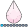## sgplot outliers

i need to create a box plot using sgplot and not disply the outliers in the graph but need to show the number of outliers(N=9) for ex.

Can any one help how can it be done

1 ACCEPTED SOLUTION

Accepted Solutionsdjrisks
Barite | Level 11

## Re: sgplot outliers

That's fine.

How many boxplots have you got? If you use the below method as an example, you could use the bandplot to try to cover up the points.

proc sgplot data=sashelp.heart;
vbox cholesterol / category=deathcause;
run;

data heart;
set sashelp.heart;
lower = 380;
upper = 600;
run;

proc sgplot data=heart;
vbox cholesterol / category=deathcause;
band x = deathcause upper = upper lower = lower / fillattrs = (color = white);
run;

5 REPLIES 5djrisks
Barite | Level 11

## Re: sgplot outliers

Hello,

This is a good reference that shows you how to create boxplots, and also include stats related to the boxplots.

http://blogs.sas.com/content/graphicallyspeaking/2015/12/23/box-plot-with-stat-table-and-markers/

I guess, you will also have to calculate the number of outliers and put it in a variable, and then plot that variable. As long as you have SAS 9.4 you should be able to use the xaxistable statements.

It would be more helpful, if you provide your example, and the version of SAS you have.

Thank you.

## Re: sgplot outliers

Yes, i am using 9.4 but not able to figure any option in sgplot which does not show the outliers in the plotdjrisks
Barite | Level 11

## Re: sgplot outliers

Okay thank you, in the VBOX statement in SGPLOT, there is the NOOUTLIERS option. Please try this. Hopefully you have it? From recollection this should hide the outiers but the range of the axis will remain the same.

## Re: sgplot outliers

Sorry for not being clear. In that case it removes all outliers but i have a range lower and upper(ex: 1.5 - 7) and if the the values are below or above these then these should not be seendjrisks
Barite | Level 11

## Re: sgplot outliers

That's fine.

How many boxplots have you got? If you use the below method as an example, you could use the bandplot to try to cover up the points.

proc sgplot data=sashelp.heart;
vbox cholesterol / category=deathcause;
run;

data heart;
set sashelp.heart;
lower = 380;
upper = 600;
run;

proc sgplot data=heart;
vbox cholesterol / category=deathcause;
band x = deathcause upper = upper lower = lower / fillattrs = (color = white);
run;

Discussion stats
• 5 replies
• 9074 views
• 2 likes
• 2 in conversation